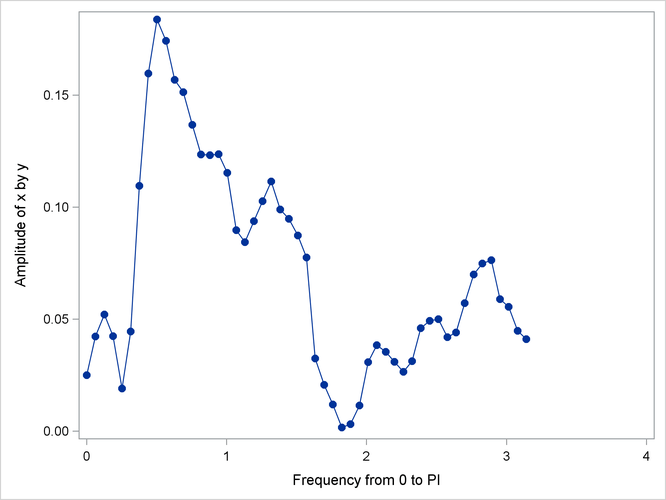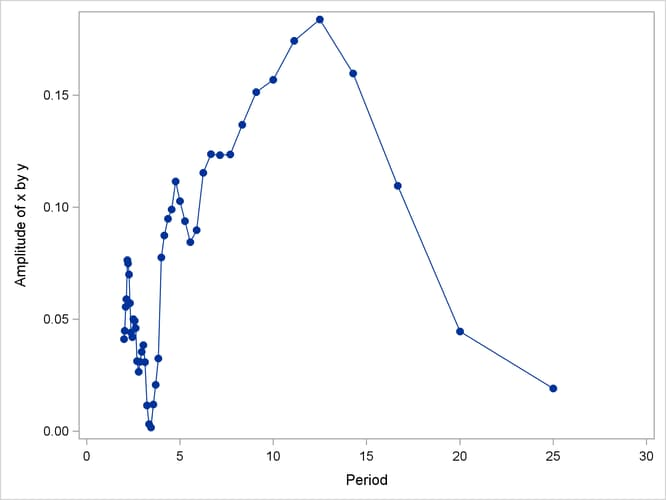The SPECTRA Procedure

Example 26.2 Cross-Spectral Analysis

This example uses simulated data to show cross-spectral analysis for two variables `X` and `Y`. `X` is generated by an AR(1) process; `Y` is generated as white noise plus an input from `X` lagged 2 periods. All output options are specified in the PROC SPECTRA statement. PROC CONTENTS shows the contents of the OUT= data set.

```data a;
xl = 0; xll = 0;
do i = - 10 to 100;
x = .4 * xl  + rannor(123);
y = .5 * xll + rannor(123);
if i > 0 then output;
xll = xl; xl = x;
end;
run;
```
```proc spectra data=a out=b cross coef a k p ph s;
var x y;
weights 1 1.5 2 4 8 9 8 4 2 1.5 1;
run;

proc contents data=b position;
run;
```

The PROC CONTENTS report for the output data set `B` is shown in Output 26.2.1.

Output 26.2.1: Contents of PROC SPECTRA OUT= Data Set

The CONTENTS Procedure

Alphabetic List of Variables and Attributes
# Variable Type Len Label
16 A_01_02 Num 8 Amplitude of x by y
3 COS_01 Num 8 Cosine Transform of x
5 COS_02 Num 8 Cosine Transform of y
13 CS_01_02 Num 8 Cospectra of x by y
1 FREQ Num 8 Frequency from 0 to PI
12 IP_01_02 Num 8 Imag Periodogram of x by y
15 K_01_02 Num 8 Coherency**2 of x by y
2 PERIOD Num 8 Period
17 PH_01_02 Num 8 Phase of x by y
7 P_01 Num 8 Periodogram of x
8 P_02 Num 8 Periodogram of y
14 QS_01_02 Num 8 Quadrature of x by y
11 RP_01_02 Num 8 Real Periodogram of x by y
4 SIN_01 Num 8 Sine Transform of x
6 SIN_02 Num 8 Sine Transform of y
9 S_01 Num 8 Spectral Density of x
10 S_02 Num 8 Spectral Density of y

The following statements plot the amplitude of the cross-spectrum estimate against frequency and against period for periods less than 25.

```proc sgplot data=b;
series x=freq y=a_01_02 / markers markerattrs=(symbol=circlefilled);
xaxis values=(0 to 4 by 1);
run;
```

The plot of the amplitude of the cross-spectrum estimate against frequency is shown in Output 26.2.2.

Output 26.2.2: Plot of Cross-Spectrum Amplitude by FrequencyThe plot of the cross-spectrum amplitude against period for periods less than 25 observations is shown in Output 26.2.3.

```proc sgplot data=b;
where period < 25;
series x=period y=a_01_02 / markers markerattrs=(symbol=circlefilled);
xaxis values=(0 to 30 by 5);
run;
```

Output 26.2.3: Plot of Cross-Spectrum Amplitude by Period